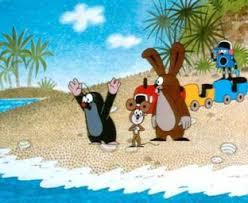# Children tickets

A ticket to the museum for children costs 10 SKK for adults and 20 SKK. 50 people came to the museum and paid 700 SKK. How many children were there?

x =  30

### Step-by-step explanation:

x+y=50
10x+20y=700

x+y=50
10•x+20•y=700

x+y = 50
10x+20y = 700

x = 30
y = 20

Our linear equations calculator calculates it.Did you find an error or inaccuracy? Feel free to write us. Thank you!

Tips for related online calculators
Do you have a linear equation or system of equations and looking for its solution? Or do you have a quadratic equation?